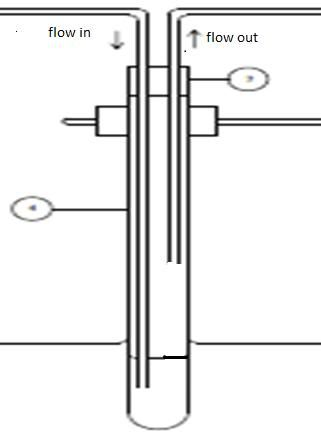1. Lets say if i have a test tube (diameter 3cm and height 30cm) seal with a rubber. in the test tube there 100ml of water = around 10 cm height. if air was flow into the test tube from bottom of the tube with flow rate around 20L/min, and after a few sec, the rubber was push out. in general i understand that the pressure inside the test tube is higher than atmospheric pressure. but i cant find any mathematical solution that relate flow rate and pressure to proof that the pressure inside the test tube is higher than outside. can anyone explain it to me.2.

3. All you need to know is the length, geometry and frictional characteristics of the outflow pipe. If the flow rate is 20l/min you can calculate the total pressure differential required to force that much flow through that particular pipe. The pressure inside the test tube is then equal to atmospheric pressure plus the calculated pressure drop.

There are many references on the web for this calculation. Don't forget entrance and exit losses.4. so the only thing i need to do is just calculate pressure loss using darcy weisbach equation based on length, geometry and frictional characteristics of the outflow pipe only and no need to consider the inlet flow of pipe?

btw what do you mean by entrance and exit losses. ?5. In addition to the frictional loss in the pipe there is a pressure drop at the pipe inlet of approx. 0.5 velocity heads and at the exit of approximately 1.0 velocity heads. In very long pipes this is often ignored, but for a short pipe it is significant.

You don't need to consider the inlet pipe at all, since all you need to know to determine the pressure in the test tube is how much pressure difference is needed for the fluid to get out.Bookmarks
 Posting Permissions
 You may not post new threads You may not post replies You may not post attachments You may not edit your posts   BB code is On Smilies are On [IMG] code is On [VIDEO] code is On HTML code is Off Trackbacks are Off Pingbacks are Off Refbacks are On Terms of Use Agreement